#### You may also like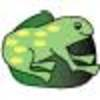### The Lily Pond

Freddie Frog visits as many of the leaves as he can on the way to see Sammy Snail but only visits each lily leaf once. Which is the best way for him to go?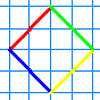### A Cartesian Puzzle

Find the missing coordinates which will form these eight quadrilaterals. These coordinates themselves will then form a shape with rotational and line symmetry.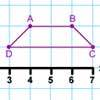### Transformation Tease

What are the coordinates of this shape after it has been transformed in the ways described? Compare these with the original coordinates. What do you notice about the numbers?

# Dotty Relationship

##### Age 7 to 11Challenge Level
On the grid below, join the two blue dots, A and B, together with a straight line.

Now join the green dots, C and D, with a straight line.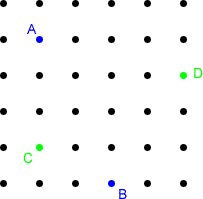At what angle do the two lines cross?

Investigate the number of squares "along" and "down" from A to B compared with the number of squares "along" and "up" from C to D. What do you notice?

Using what you have found out, can you draw lines that are perpendicular (at $90^{\circ}$) to the lines drawn below?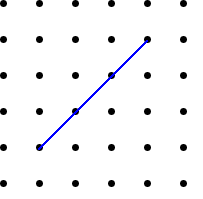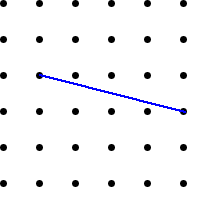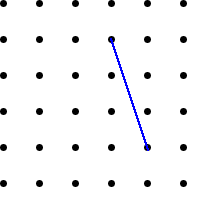Is there only one solution each time?

Do the two lines have to be the same length? Why or why not?